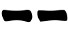# I Ching quipu

For a long time I've thought about a way to cast I Ching hexagrams using knotted strings.

I've tried many different designs and I'm still not 100% satisfied. However this quipu (yes, I know it's not a real quipu) is so simple to build, easy to handle and pleasant to the eye that I decided to present it here.

To see how the two strings are tied togther, just enlarge the picture, it should be pretty straightforward to replicate. In the center there is a triple fisherman's knots, at each end there's a figure 8 knot tightened.

A nice feature of this object is that it can be used to generate lines with different probabilities.

#### Three Coins Probabilities

1. Pick (without looking) one of the knots at the end of the strings;
2. If it's red, write down 3; if it's black, write down 2;
3. Repeat steps 1-2 other two times and sum up the numbers;
4. Draw the line according the following table:
 6 7 8 95. Repeat steps 1-4 other five times drawing the resulting lines from the bottom to the top of the hexagram.
Choosing one of the string ends is equivalent to throwing one of the coin and the probabilities are:
Prob(6) = Prob(9) = 1/8 = 12.5%
Prob(8) = Prob(7) = 3/8 = 37.5%
Prob(yin) = Prob(yang) = 1/2
Note how each pick corresponds to one of the coins in the three coins method.

#### Yarros Stalks Probabilities

1. Pick (without looking) one of the knots at the end of the strings;
2. If it's black, coming out the black knot write down 2, otherwise write 3;
3. Pick (without looking) one of the knots at the end of the strings;
4. If it's red write down 3, if it's black, write down 2;
5. Repeat ste steps 3-4 again and sum up the numbers;
6. Draw the line according the following table:
 6 7 8 97. Repeat steps 1-6 other five times drawing the resulting lines from the bottom to the top of the hexagram.
The choice in step 1 will give 2 with probability 1/ and 3 with probability 3/4 , the other steps will give 2 with probability 2/4 and 3 with probability 2/4, meaning that the probabilities are:
Prob(6) = 1/16 = 6.25%
Prob(8) = 7/16 = 43.75%
Prob(7) = 5/16 = 31.25%
Prob(9) = 3/16 = 18.75%
Prob(yin) = Prob(yang) = 1/2

Note how the first pick corresponds to the first split of the 49 stalks and the others to the subsequent splits.

#### Equal Probabilities

1. Pick (without looking) one of the knots at the end of the strings;
• If it's black, coming out the red knot, write down 6
• If it's black, coming out the black knot, write down 8
• If it's red, coming out the red knot, write down 7
• If it's red, coming out the black knot, write down 9
2. Draw the line according the following table:
 6 7 8 93. Repeat steps 1-2 other five times drawing the resulting lines from the bottom to the top of the hexagram.
Each line has the same probability to be drawn:
Prob(6) = 1/4 = 25%
Prob(8) = 1/4 = 25%
Prob(7) = 1/4 = 25%
Prob(9) = 1/4 = 25%
Prob(yin) = Prob(yang) = 1/2

#### Variations

Same principle but with a white string instead of a red one. The central knot will help handling the quipu while casting the lines.This work is licensed under a Creative Commons Attribution-NonCommercial 4.0 International License.